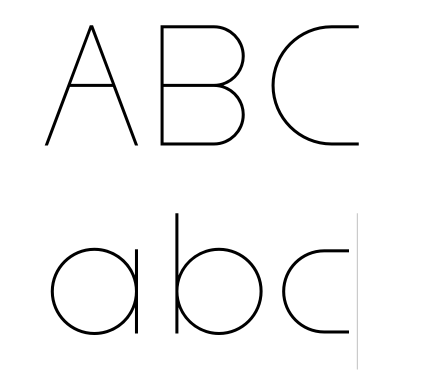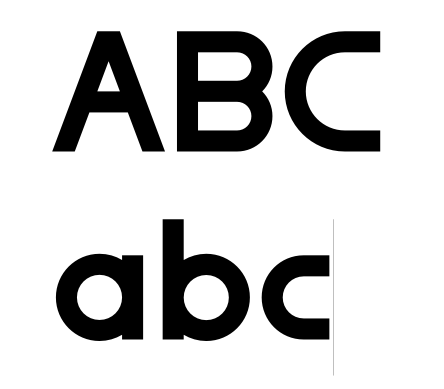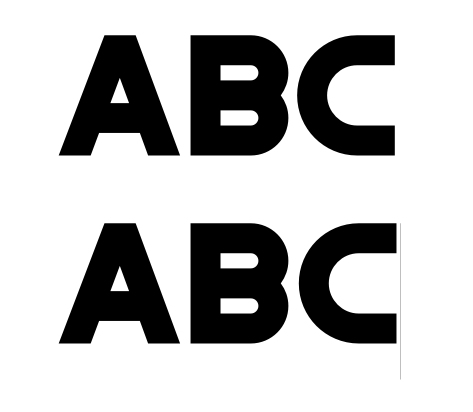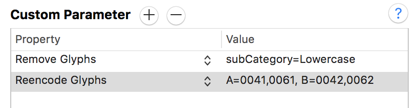# Small letters as capital - with kerning

Hi, I have created font but just with small letters. I don’t need capital letters but I want if someone type capital on keyboard have also text. Is there any chance to set small letters as capital? Maybe any copping ? But I need also copy kerning.

Put components of each lowercase letter into the relevant cap slots. Then assign the cap versions to the relevant kerning classes.

Thanks for help, how to copy these components ?

I need also have solved situation like “Ava”, “aVa”, “avA” etc.

ok, but it don’t solve the problem with kerning. i copied even all kerning groups, like in small letters but kerning don’t work in capital

You nee to have class kerning and the same classes in lower and upper case. glyphsapp.com/tutorials/kerning

Thanks, added the same classes helped. But last time I created second master and problem appeared again. If I delete first or second master - again work.

What do you mean by that? Can you explain the steps 1, 2, 3…?

Hi, I am also developing an all-caps font and would like to share the simple equations for the three basic European alphabets. I hope this will save people the trouble of typing the names by hand.Latin
A=a B=b C=c D=d E=e F=f G=g H=h I=i J=j K=k L=l M=m N=n O=o P=p Q=q R=r S=s T=t U=u V=v W=w X=x Y=y Z=z

Greek
Alpha=alpha Beta=beta Gamma=gamma Delta=delta Epsilon=epsilon Zeta=zeta Eta=eta Theta=theta Iota=iota Kappa=kappa Lambda=lambda Mu=mu Nu=nu Xi=xi Omicron=omicron Pi=pi Rho=rho Sigma=sigma Tau=tau Upsilon=upsilon Phi=phi Chi=chi Psi=psi Omega=omega

(The lc letter sigma has two forms, use Sigma=sigmafinal if you want to repeat the caps version of the letter. As far as i gather there is no uppercase version of sigmafinal)

Cyrillic
A-cy=a-cy Be-cy=be-cy Ve-cy=ve-cy Ge-cy=ge-cy De-cy=de-cy Ie-cy=ie-cy Io-cy=io-cy Zhe-cy=zhe-cy Ze-cy=ze-cy Ii-cy=ii-cy Iishort-cy=iishort-cy Ka-cy=ka-cy El-cy=el-cy Em-cy=em-cy En-cy=en-cy O-cy=o-cy Pe-cy=pe-cy Er-cy=er-cy Es-cy=es-cy Te-cy=te-cy U-cy=u-cy Ef-cy=ef-cy Ha-cy=ha-cy Che-cy=che-cy Tse-cy=tse-cy Sha-cy=sha-cy Shcha-cy=shcha-cy Ia-cy=ia-cy Softsign-cy=softsign-cy Hardsign-cy=hardsign-cy Yeru-cy=yeru-cy Ereversed-cy=ereversed-cy Iu-cy=iu-cy

Checked&working.

1 Like

How to solve kerning in a family of lower-/uppercase and only uppercase?
My font consists of three masters, #1 & 2 are lower-/uppercase and #3 is only uppercase:Because the kerning classes affect all three masters I got a problem: the kerning classes for the minuscules from masters 1 & 2 are obviously useless in the all caps master. Is there a smart way to make an “exception kerning class” for the capital minuscules in master three? Or to copy the kerning data from the caps to the minuscules?

Do not put UC shapes in the LC slots. Rather, in the instances that do not have LC letters, use a Remove Glyphs and a Reencode Parameter.

• In Remove Glyphs, you can use a `subCategory=Lowercase` wildcard.
• In Reencode Glyphs, you add lines containing `glyphname=uppercaseunicode,lowercaseunicode`, e.g., `A=0041,0061` and `B=0042,0062` and so on.1 Like

Cool, thank you! I really knew there will be such a clever way … It works well but not with the SC diacriticals. I tried the Remove Glyphs Parameter with subCategory=Lowercase wildcard and also all SC as a simple string (except germandbls and fi fl).

In the exported font the LC diacritics rest empty. Could you give me another hint please?

You need to add the glyph names. Select all of them in font view and right click and Copy Glyphs Names > “One per Line”. Then paste that in the custom parameter.

1 Like

Yes, now every thing works fine! Thank you both. That is a good day today!

1 Like